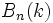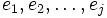# Borel subgroup is self-normalizing in general linear group

This article gives the statement, and possibly proof, of a particular subgroup or type of subgroup (namely, Borel subgroup in general linear group (?)) satisfying a particular subgroup property (namely, Self-normalizing subgroup (?)) in a particular group or type of group (namely, General linear group (?)).

## Statement

Let$k$ be any field, and$GL_n(k)$ denote the General linear group (?) of$n \times n$ matrices over$k$. The Borel subgroup$B_n(k)$ is the subgroup of upper-triangular invertible$n \times n$ matrices.$B_n(k)$ is a Self-normalizing subgroup (?) inside$GL_n(k)$.

## Related facts

### For finite fields

If$k$ is the finite field of order$q$ where$q$ is a power of a prime$p$, the Borel subgroup$B_n(k)$ equals the normalizer of a$p$-Sylow subgroup of$GL_n(k)$: the subgroup of upper-triangular matrices with$1$s on the diagonal. The normalizer of any Sylow subgroup in a finite group is self-normalizing; in fact, it is an abnormal subgroup. Further information: Sylow normalizer implies abnormal

## Proof

### Hands-on proof

The proof relies on a somewhat different interpretation of$B_n(k)$. Let$e_1, e_2, \dots, e_n$ denote the standard basis for$k^n$. Then, the standard flag is an ascending chain of subspaces:$V_0 \le V_1 \le V_2 \le \dots \le V_n$
where the subspace$V_j$ is the span of the vectors$e_1,e_2, \dots, e_j$. Then,$B_n(k)$ is the subgroup of$GL_n(k)$ comprising those linear transformations that preserve this flag. In other words,$A \in B_n(k)$ if and only if the flag corresponding to the basis$Ae_1, Ae_2, \dots, Ae_n$ is the same as the flag corresponding to the basis$e_1, e_2, \dots, e_n$.
Now, given any$A \in GL_n(k)$, the subgroup$AB_n(k)A^{-1}$ is precisely the subgroup of$GL_n(k)$ that stabilizes the flag of$Ae_1, Ae_2, \dots, Ae_n$. Thus, for$A$ to normalize$B_n(k)$ we require that the linear transformations stabilizing the flag of$e_1,e_2, \dots, e_n$ are the same as the linear transformations of the flag$Ae_1, Ae_2, \dots, Ae_n$. We can now prove, through an inductive argument, that this forces the flags for the two bases to be the same, forcing$A \in B_n(k)$.